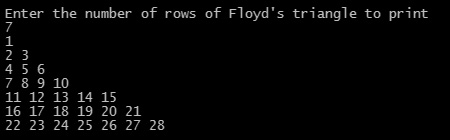# C Program to Create Floyd's Triangle

A right-angled triangular array of natural numbers is called Floyd triangle.

Building Floyd's triangle in C programming is quite easy, but you must have the understanding of how for loop works.

Program:
``````#include <stdio.h>

int main()
{
int n, i,  c, a = 1;

printf("Enter the number of rows of Floyd's triangle to print\n");
scanf("%d", &n);

for (i = 1; i <= n; i++)
{
for (c = 1; c <= i; c++)
{
printf("%d ",a);
a++;
}
printf("\n");
}

return 0;
}
``````
Output of program:Explanation:

This program is used to print the right-angled Floyd's triangle. For this you just need to understand the working of for-loop. So first of all, you have to include the stdio header file using the "include" preceding by # which tells that the header file needs to be process before compilation, hence named preprocessor directive.

Then the main() function is declared with return type as integer. Now you have to declare four integer type variables name as 'n', 'i', 'c' and 'a' and assign the value of 'a' as 1. Then the printf() function is used for displaying the message -  "Enter the number of rows of Floyd's triangle to print: ". The scanf() function then fetches the value from the user and stores it in variable n.

Now, you have to use 2 for loops in the form of nested for. First for loop will count using the variable i as 1 and checks the condition i <= n. Again the second nested for loop goes from c=1 till c <=i.

Then the printf() function is used within nested for to print all the numbers and increments the value of 'a' by 1. After the inner loop gets executed, the printf() will take the printing cursor to the next line using the escape sequence character '\n'